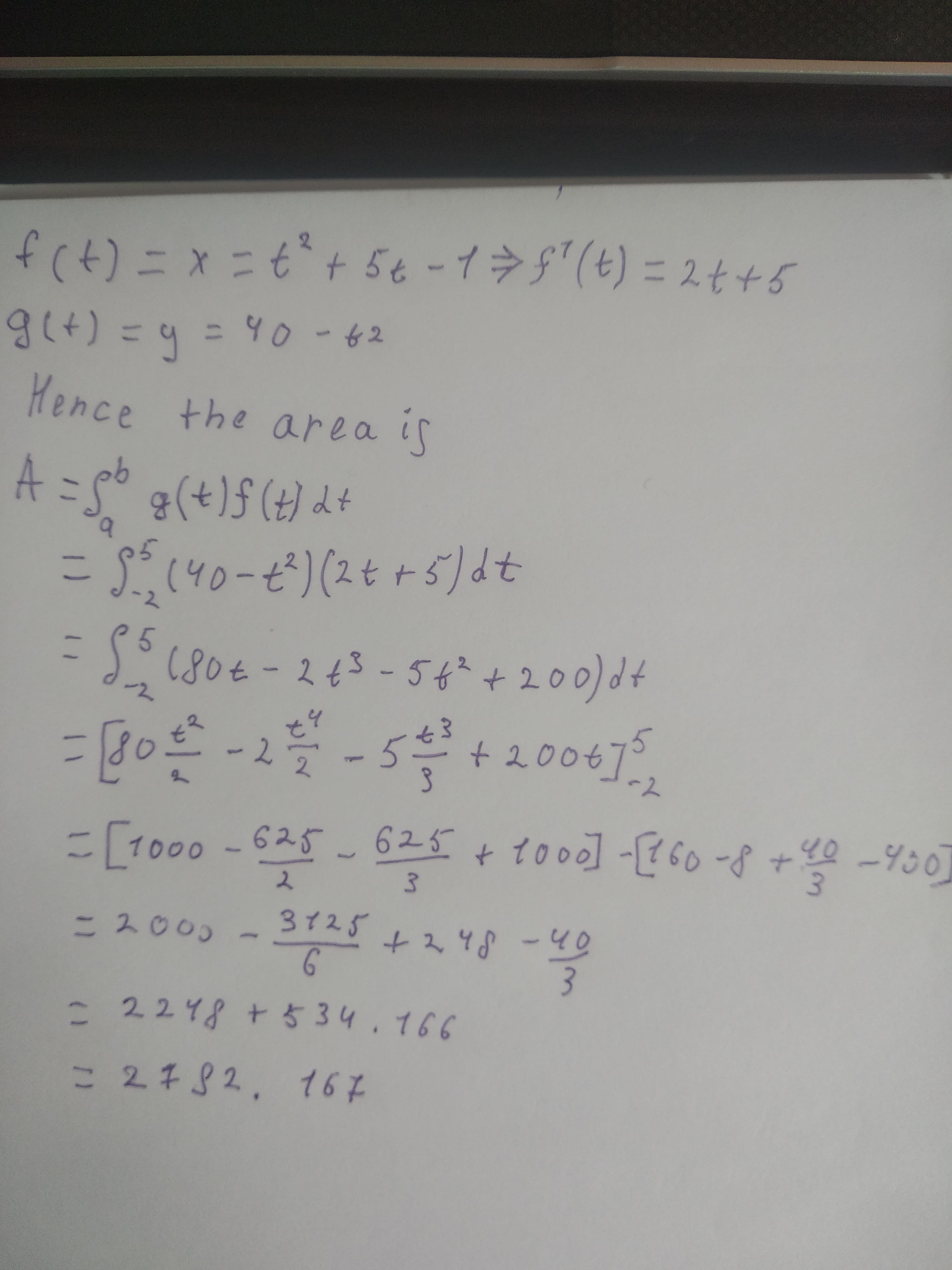# Determine the area of the region below the parametric curve given by the set of parametric equations. For each problem you may assume that each curveReggie 2020-11-06 Answered
Determine the area of the region below the parametric curve given by the set of parametric equations. For each problem you may assume that each curve traces out exactly once from right to left for the given range of t. For these problems you should only use the given parametric equations to determine the answer. 1.$x={t}^{2}+5t-1y=40-{t}^{2}-2\le t\le 5$ 2.$x=3co{s}^{2}\left(t\right)—si{n}^{2}\left(t\right)y=6+cos\left(t\right)-\frac{\pi }{2}\ne t\le 0$ 3.$x={e}^{\frac{1}{4}t}—2y=4+{e}^{\frac{1}{4t}}—{e}^{\frac{1}{4}t}-6\le t\le 1$
You can still ask an expert for help

• Questions are typically answered in as fast as 30 minutes

Solve your problem for the price of one coffee

• Math expert for every subject
• Pay only if we can solve itsaiyansruleA

Given: As per our honor code, we are answering only the first question. The parametric equations are $x={t}^{2}+5t-1,y=40-{t}^{2},-2\le t\ne 5$. We have to find the area of the region bounded by these parametric equations. Concept Used: If the parametric equations are $x=f\left(t\right),y=g\left(t\right)$ and
$a\le t\le b$ then the area of the bounded region is given by $A={\int }_{a}^{b}g\left(t\right)f\left(t\right)dt$ Calculating: